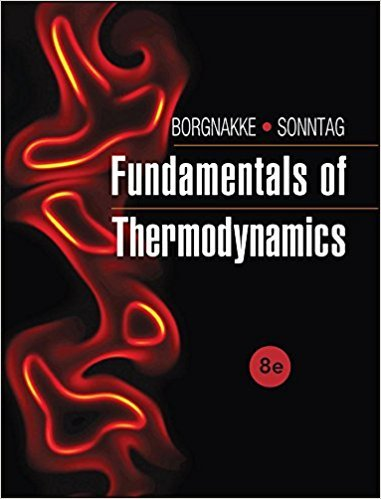×
Log in to StudySoup
Get Full Access to Fundamentals Of Thermodynamcs - 8 Edition - Chapter 3 - Problem 5cgp
Join StudySoup for FREE
Get Full Access to Fundamentals Of Thermodynamcs - 8 Edition - Chapter 3 - Problem 5cgp

Already have an account? Login here
×
Reset your password

# In Fig. P3.5, CV A is the mass inside a piston/ cylinder,ISBN: 9781118131992 58

## Solution for problem 5CGP Chapter 3

Fundamentals of Thermodynamcs | 8th Edition

• Textbook Solutions
• 2901 Step-by-step solutions solved by professors and subject experts
• Get 24/7 help from StudySoup virtual teaching assistantsFundamentals of Thermodynamcs | 8th Edition

4 5 1 238 Reviews
16
5
Problem 5CGP

Problem 5CGP

In Fig. P3.5, CV A is the mass inside a piston/ cylinder, and CV B is the mass plus the piston outside, which is the standard atmosphere. Write the energy equation and work term for the two CVs, assuming we have a nonzero Q between state 1 and state 2.

FIGURE P3.5Step-by-Step Solution:

Solution 5 CGP:

Step 1 of 2:-

Here we have to write down the energy equation and work terms for the two CVs.

The process for A is,.

The energy equation will be,The work can be calculated as,Step 2 of 2

##### ISBN: 9781118131992

This full solution covers the following key subjects: mass, state, piston, assuming, cylinder. This expansive textbook survival guide covers 7 chapters, and 1462 solutions. The answer to “In Fig. P3.5, CV A is the mass inside a piston/ cylinder, and CV B is the mass plus the piston outside, which is the standard atmosphere. Write the energy equation and work term for the two CVs, assuming we have a nonzero Q between state 1 and state 2.FIGURE P3.5” is broken down into a number of easy to follow steps, and 51 words. Fundamentals of Thermodynamcs was written by and is associated to the ISBN: 9781118131992. This textbook survival guide was created for the textbook: Fundamentals of Thermodynamcs , edition: 8. Since the solution to 5CGP from 3 chapter was answered, more than 342 students have viewed the full step-by-step answer. The full step-by-step solution to problem: 5CGP from chapter: 3 was answered by , our top Engineering and Tech solution expert on 08/03/17, 05:05AM.

Unlock Textbook Solution

Enter your email below to unlock your verified solution to:

In Fig. P3.5, CV A is the mass inside a piston/ cylinder,